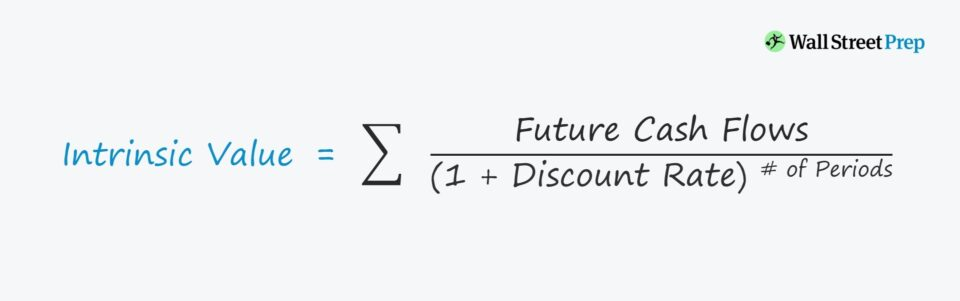Welcome to Wall Street Prep! Use code at checkout for 15% off.# Intrinsic Value

Guide to Understanding the Intrinsic Value Concept## How to Calculate Intrinsic Value (Step-by-Step)

The premise of intrinsic value states that how much an asset is worth can be derived from assessing the asset internally.

For instance, a company’s share price can be approximated by assessing the underlying fundamentals:

In the context of corporate valuation, the intrinsic value of a company is estimated from its future cash flows, growth potential, and risk. Thus, the foundation of a DCF valuation model is the 3-statement financial model.#### Build a 3-Statement Financial Model (Free Course)Submitting...

## Intrinsic Value Formula

The formula for calculating the intrinsic value states the asset’s estimated worth is a function of its future cash flows, which must be discounted to the present date.

The expected cash flows of the asset are each discounted, and the sum of those cash flows represents the asset’s intrinsic value.

Intrinsic Value = Σ CF / (1 + r) ^ t

Where:

## Intrinsic Value Method – Discounted Cash Flow (DCF) Model

The discounted cash flow model (DCF) approach calculates the present value (PV) of the company’s expected cash flows (i.e. discounted to the present date), which is the estimated value of the company.

Here, all the future cash flows (CF) of the company are discounted using an appropriate discount rate (r) that factors in risk – and then adds all the discounted cash flows together.

Therefore, the intrinsic valuation is a function of the future free cash flows – either FCFF or FCFE – expected to be generated by the company’s operations.

Each DCF model relies significantly on discretionary assumptions.

While all assumptions are subjective, if the model assumptions are completely baseless, the estimated value of the company will be far off from its intrinsic (“true”) value.

• > Current Share Price → Undervalued – Potential Buy
• = Current Share Price → “Correct” Market Pricing
• < Current Share Price → Overvalued – Potential Short-Sell

## Intrinsic Value Method – Dividend Discount Model (DDM)

Another intrinsic valuation method is the dividend discount model (DDM), although the DDM is not used as frequently as the DCF.

The dividend discount model (DDM) values a company based on the present value (PV) of its future dividends, with assumptions regarding the dividend amount and growth rate.

The intuition behind the DDM is similar to the DCF, however, the major difference is that dividends are used as the cash flows.

Under the DDM, the dividends issued by a company are assumed to be representative of the company’s financial health and future outlook.

The intrinsic value – considering how the obtained valuation is largely independent of market pricing – can uncover undervalued investment opportunities for investors to profit from the mispricing.Step-by-Step Online Course

### Everything You Need To Master Financial Modeling

Enroll in The Premium Package: Learn Financial Statement Modeling, DCF, M&A, LBO and Comps. The same training program used at top investment banks.Inline FeedbacksLearn Financial Modeling Online

Everything you need to master financial and valuation modeling: 3-Statement Modeling, DCF, Comps, M&A and LBO.

X

The Wall Street Prep Quicklesson Series

7 Free Financial Modeling Lessons

Get instant access to video lessons taught by experienced investment bankers. Learn financial statement modeling, DCF, M&A, LBO, Comps and Excel shortcuts.